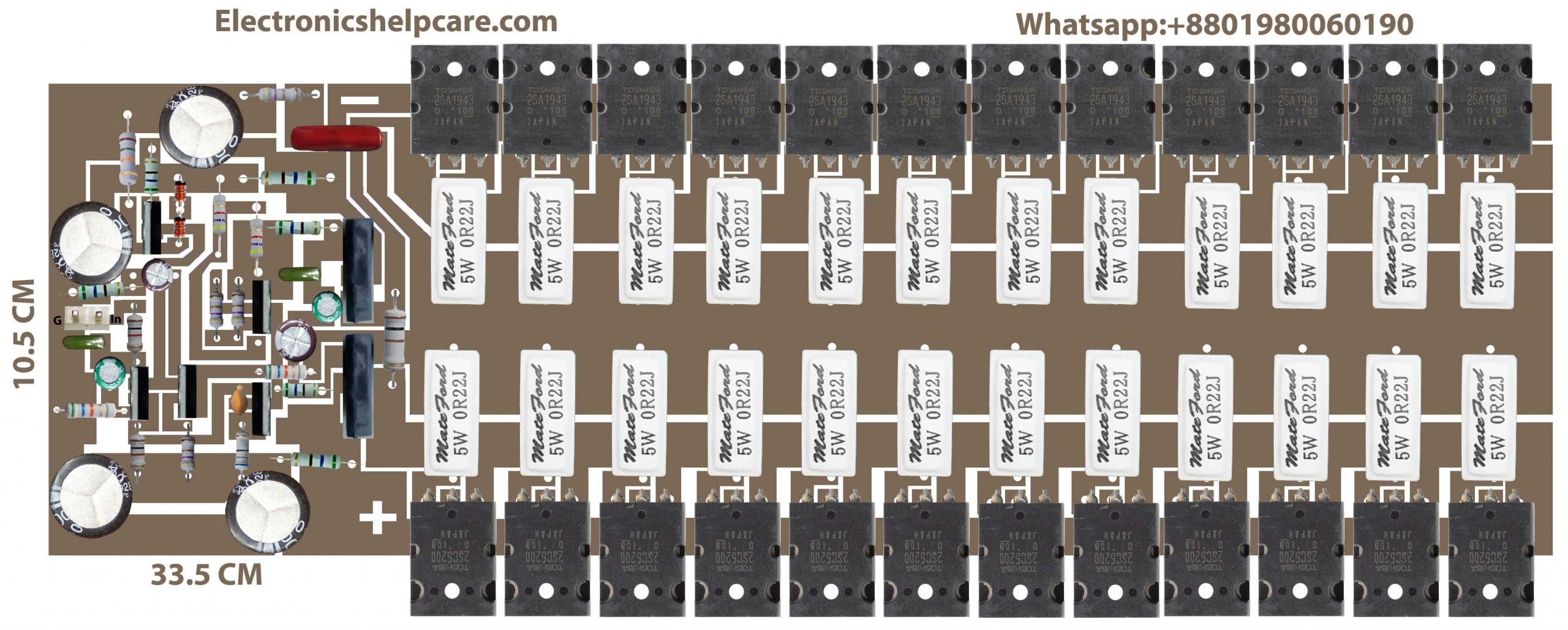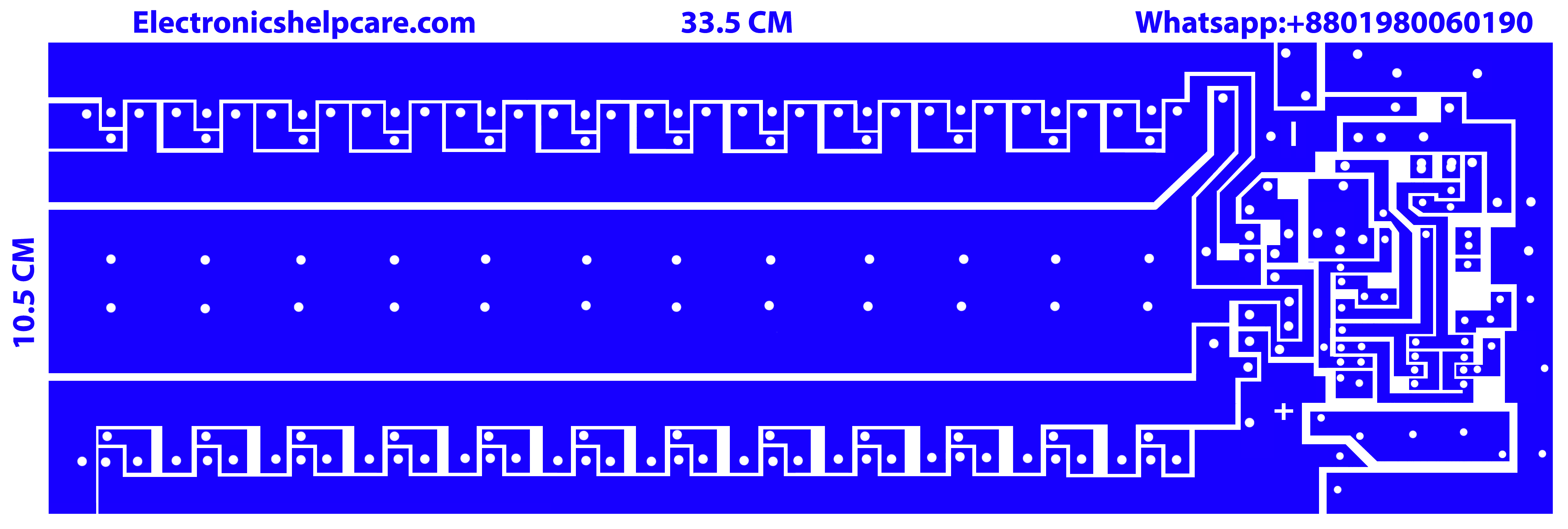Breaking News

# 2sc5200 and 2sa1943 transistor amplifier circuit diagram

## 2sc5200 and 2sa1943 transistor amplifier circuit diagram:

2sc5200 and 2sa1943 transistor amplifier circuit diagram. This is PNP and NPN amplifier circuit diagram. We used 2sc5200 and 2sa1943. 2sc5200 is NPN transistor and 2sa1943 is PNP transistor. We can use maximum 230 volt and 1.5 ampere. But here in this circuit diagram we can use maximum 50 voltage. The voltage depends on various circuits. This circuit is for maximum 50 voltage. We can use maximum 1.5 ampere for 1 transistor.

2sc5200 and 2sa1943 transistor amplifier circuit diagram2sc5200 and 2sa1943 transistor amplifier circuit diagram.jpg2sc5200 and 2sa1943 transistor amplifier circuit diagramHow to calculate watts?

We know that voltage X ampere = watts, so if we use 2 transistors we have to use 35-0-35 voltage and 3 ampere because 1 transistor can take 1.5 ampere. According to watt calculation rules 35 X 6=210 watts. Here we have a question that why 6 ampere? Why not 3 ampere? Because here voltage is dual. According to rules; voltage will be same 35 but ampere will be double 3+3=6. Our voltage start from ground to 35 both side positive and negative and both side have 3 amp. In this circuit taking 35 volts and  amperes from both side 3+3=6.

Using voltage and amperes for this 24 transistors circuit

In this circuit we can use 50 voltage and 24 X 1.5=36 amperes maximum. But we use 50 voltage and 20 amperes for this circuit. So,50 X 20=1000 watts. We have to use 2 amplifier circuit diagram for stereo sound. 1000+1000=2000 watts amplifier we are going to make.

Using best transformer:                                                                                                                                                  Transformer is the most important to getting right watts. If we don’t use good quality of transformer then we can not get good watts.  If we use 2 circuits we have to use dual side center tap transformer for best performance.

 Symbol Parameter Conditions MAX VCBO Collector-base voltage Open emitter +230V VCEO Collector-emitter voltage Open base +230V VEBO Emitter-base voltage Open collector +5V IC Collector current +15 A IB Base current +1.5 A PC Collector power dissipation TC=25℃ 150 W Tj Junction temperature 150 ℃ Tstg Storage temperature -55~150 ℃

 Symbol Parameter Conditions MAX VCBO Collector-base voltage Open emitter -230V VCEO Collector-emitter voltage Open base -230V VEBO Emitter-base voltage Open collector -5V IC Collector current -15 A IB Base current -1.5 A PC Collector power dissipation TC=25℃ 150 W Tj Junction temperature 150 ℃ Tstg Storage temperature -55~150 ℃